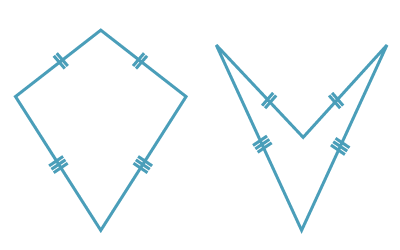## Kites##### Definition of a kite
• A kite is a quadrilateral with two pairs of adjacent sides equal.
• A kite may be convex or non-convex.
##### Properties of a kite
• The angles opposite the axis of a kite are equal.
• The axis of a kite bisects the vertex angles through which it passes.
• The axis of a kite is the perpendicular bisector of the other diagonal.
##### Test for a kite

A quadrilateral is a kite if:

• one diagonal bisects the vertex angles through which it passes, or
• one diagonal is the perpendicular bisector of the other diagonal.